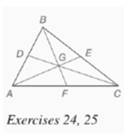Chapter 7.CR, Problem 25CRElementary Geometry For College St...

7th Edition
Alexander + 2 others
ISBN: 9781337614085

Solutions

Chapter
SectionElementary Geometry For College St...

7th Edition
Alexander + 2 others
ISBN: 9781337614085
Textbook Problem

Given: Δ A B C (in Exercise 24 ) with medians A E , ¯ C D , ¯ B F ¯ A G = 2 x + 2 y , G E = 2 x − y B G = 3 y + 1 , G F = xFind: B F and A E

To determine

To find: BF and AE.

Explanation

Given:

The triangle ABC with medians AE¯,CD¯,BF¯, AG=2x+2y,GE=2xy,BG=3y+1,GF=x

The point G divides the median in the ration 2:1.

Calculation:

From the figure,

AGGE=212x+2y2xy=212x+2y=4x2y2x4y=0x2y=0 ...(1)

From the figure,

BGGF=213y+1x=213y+1=2x2x3y=1

Still sussing out bartleby?

Check out a sample textbook solution.

See a sample solution

The Solution to Your Study Problems

Bartleby provides explanations to thousands of textbook problems written by our experts, many with advanced degrees!

Get Started

Radical Expressions Simplify the expression. 51. (a) 9a3+a (b) 16x+x5

Precalculus: Mathematics for Calculus (Standalone Book)

Find f in terms of g. f(x) = g(x2)

Single Variable Calculus: Early Transcendentals

Using tan2 x = sec2 x − 1, ∫ tan3 x dx =

Study Guide for Stewart's Single Variable Calculus: Early Transcendentals, 8th

The area of the shaded region is given by:

Study Guide for Stewart's Multivariable Calculus, 8th